# Verbal Reasoning - Dice

## Why Verbal Reasoning Dice?

In this section you can learn and practice Verbal Reasoning Questions based on "Dice" and improve your skills in order to face the interview, competitive examination and various entrance test (CAT, GATE, GRE, MAT, Bank Exam, Railway Exam etc.) with full confidence.

## Where can I get Verbal Reasoning Dice questions and answers with explanation?

IndiaBIX provides you lots of fully solved Verbal Reasoning (Dice) questions and answers with Explanation. Solved examples with detailed answer description, explanation are given and it would be easy to understand. All students, freshers can download Verbal Reasoning Dice quiz questions with answers as PDF files and eBooks.

## Where can I get Verbal Reasoning Dice Interview Questions and Answers (objective type, multiple choice)?

Here you can find objective type Verbal Reasoning Dice questions and answers for interview and entrance examination. Multiple choice and true or false type questions are also provided.

## How to solve Verbal Reasoning Dice problems?

You can easily solve all kind of Verbal Reasoning questions based on Dice by practicing the objective type exercises given below, also get shortcut methods to solve Verbal Reasoning Dice problems.

### Exercise :: Dice - Dice 1

1.

Which symbol will be on the face opposite to the face with symbol * ?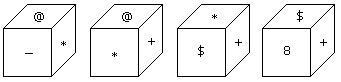A. @ B. \$ C. 8 D. +

Explanation:

The symbols of the adjacent faces to the face with symbol * are @, -, + and \$. Hence the required symbol is 8.

2.

Two positions of dice are shown below. How many points will appear on the opposite to the face containing 5 points?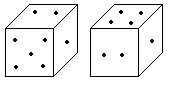A. 3 B. 1 C. 2 D. 4

Explanation:

In these two positions one of the common face having 1 point is in the same position. Therefore according to rule (2). There will be 4 points on the required face.

3.

Which digit will appear on the face opposite to the face with number 4?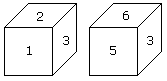A. 3 B. 5 C. 6 D. 2/3

Explanation:

Here the common faces with number 3, are in same positions. Hence 6 is opposite to 2 and 5 is opposite to 1. Therefore 4 is opposite to 3.

4.

Two positions of a dice are shown below. Which number will appear on the face opposite to the face with the number 5?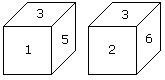A. 2/6 B. 2 C. 6 D. 4

Explanation:

According to the rule no. (3), common faces with number 3, are in same positions. Hence the number of the opposite face to face with number 5 will be 6.

5.

How many points will be on the face opposite to in face which contains 2 points?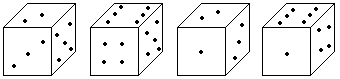A. 1 B. 5 C. 4 D. 6